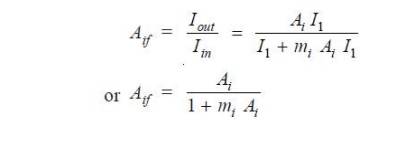Home | | Electronic Circuits II | Current Gain with Negative Current Feedback

# Current Gain with Negative Current Feedback

This equation looks very much like that for the voltage gain of negative voltage feedback amplifier.

Current Gain with Negative Current Feedback

Referring, we have,

Iin = I1 + If = I1 + mi Iout

But Iout = Ai I1, where Ai is the current gain of the amplifier without feedback.

Iin = I1 + mi Ai I1 (ä Iout = Ai I1)

Current gain with negative current feedback isThis equation looks very much like that for the voltage gain of negative voltage feedback amplifier. The only difference is that we are dealing with current gain rather than the voltage gain.

The following points may be noted carefully :

(i)  The current gain of the amplifier without feedback is Ai. However, when negative current feedback is applied, the current gain is reduced by a factor (1 + mi Ai).

(ii)       The feedback fraction (or current attenuation) mi has a value between 0 and 1.

(iii) The negative current feedback does not affect the voltage gain of the amplifier.

Study Material, Lecturing Notes, Assignment, Reference, Wiki description explanation, brief detail
Electronic Circuits : Feed Back Amplifiers : Current Gain with Negative Current Feedback |